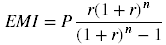×

# EMI Calculator

 Loan Amount (\$): Annual Interest Rate: % Loan Tenure: months

The EMI Calculator is used to calculate the EMI (Equated Monthly Installment) and find out how much you need to pay every month towards your loan repayment.

## FAQ

EMI stands for equated monthly installment, which is a fixed payment owed each month on a property mortgage or other loan.

The EMI calculation formula is as follows:where:
P = principal loan amount
r = annual interest rate / 12
n = number of monthly installments

## All of Our Miniwebtools (Sorted by Name):

•{{name}}
{{title}}

Miniwebtool

If you like EMI Calculator, please consider adding a link to this tool by copy/paste the following code:

Watch Videos: